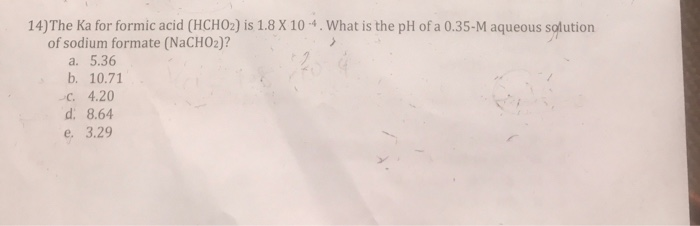# Question & Answer: The Ka for formic acid (HCHO_2) is 1.8 times 10^-4. What is the pH of a 0.35-M aqueous…..The Ka for formic acid (HCHO_2) is 1.8 times 10^-4. What is the pH of a 0.35-M aqueous solution of sodium formate (NaCHO_2)? a. 5.36 b. 10.71 c. 4.20 d. 8.64 e. 3.29

K a ( formic acid ) = 1.8*10-4, concentration of NaCHO2 = .35M

In aqueous media CHO2 ion is in equilubria, HCOO + H2 HCOOH + OH initial concentration of HCOOH and OH are 0,but later x amount of HCOOH and x amount of OH will form.

Don't use plagiarized sources. Get Your Custom Essay on
Question & Answer: The Ka for formic acid (HCHO_2) is 1.8 times 10^-4. What is the pH of a 0.35-M aqueous…..
GET AN ESSAY WRITTEN FOR YOU FROM AS LOW AS \$13/PAGE

HCOO + H2 HCOOH +OH

.35 0 0

.35-x x x

then Kb is defined like, Kb =

Kb =

Ka*Kb = 10-14 , Kb = 10-14/1.8*10-4

Kb =5.56 *10-11 =  , since  Kb = /.35

then  =4.41*10-6 =  , pOH = -log    = 5.35

pH = 14 – pOH

= 8.6 , option d is correct